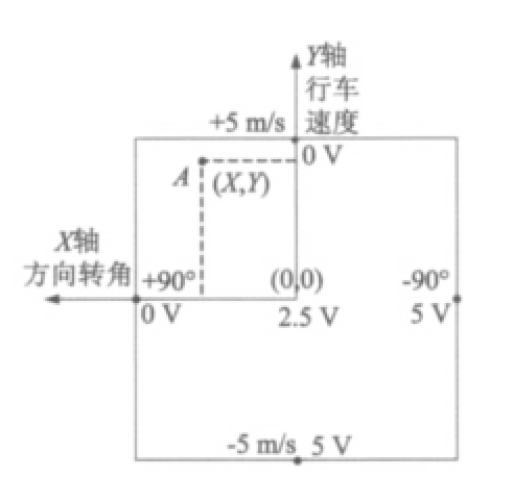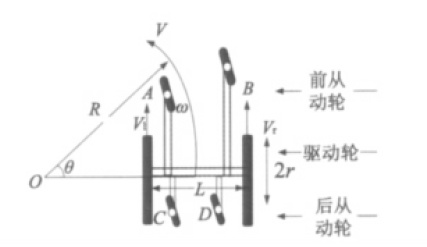# 轮椅的两轮差速转向控制策略（智能轮椅）

3 篇文章 0 订阅

##### 手柄信号说明##### 算法过程

V = 5 ∗ 2.5 − Y 2.5 V = 5 * \frac{2.5 - Y}{2.5}
θ = 90 ° ∗ 2.5 − X 2.5 \theta= 90° * \frac{2.5-X}{2.5}V = ( w r + w l ) ∗ r 2 V=(w_{r} + w_{l}) * \frac{r}{2}
R = L 2 ∗ w r + w l w r − w l R= \frac{L}{2} * \frac{w_{r} + w_{l}}{w_{r} - w_{l}}

w r = ( 2 R + L ) ∗ V 2 R r w_{r} = \frac{(2R+L)*V}{2Rr}
w l = ( 2 R − L ) ∗ V 2 R r w_{l} = \frac{(2R-L)*V}{2Rr}
w = ( w r − w l ) r L w = \frac{(w_{r}-w_{l})r}{L}

##### 轮椅的三种运动状态
1. 当θ = 0， V≠0时，即要求电动轮椅作速度为V的直线运动，此时轮椅车体的质心线速度 V = V r = V l V= V_{r} = V_{l} ，转弯半径R = ∞。这时，左右电机应该实现转速跟随。两轮速度如下： w r = w l = V r w_{r} = w_{l} = \frac{V}{r}
2. 当θ ≠ 0， V≠0时，即要求电动轮椅转弯运行，转弯半径R在0与 ∞之间。这时，左右电机应该实现转速跟随。两轮速度如下： w r = ( 2 R + L ) ∗ V 2 R r w_{r} = \frac{(2R+L)*V}{2Rr}
w l = ( 2 R − L ) ∗ V 2 R r w_{l} = \frac{(2R-L)*V}{2Rr}
3. 当θ ≠ 0， V=0时，即要求电动轮椅作回转半径为零的原地回转，运动控制器需要保证左右轮的线速度 V l = − V w = V m ( V m 由 系 统 的 机 械 特 性 选 定 ） V_{l} = -V_{w} = V_{m}(V_{m}由系统的机械特性选定） ，两轮转速如下： w r = w l = V m r w_{r} = w_{l} = \frac{V_{m}}{r}
由以上分析可知，通过操作手柄信号处理算，可以得到左右电机转速和转向的控制信号，进而实现轮椅的各个动作。

12-28
10-24850509-1361
07-075560
08-313万+
02-016243
02-284937
02-275270
12-101278
08-02
03-0864
06-032746
02-232708
09-121345点击重新获取扫码支付余额充值